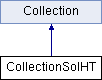ParM  parm A molecular dynamics library
CollectionSolHT Class Reference

#include <collection.hpp>

Inheritance diagram for CollectionSolHT:## Public Member Functions

CollectionSolHT (sptr< Box > box, sptr< AtomGroup > atoms, const flt dt, const flt damping, const flt desired_temperature, vector< sptr< Interaction > > interactions=vector< sptr< Interaction > >(), vector< sptr< StateTracker > > trackers=vector< sptr< StateTracker > >(), vector< sptr< Constraint > > constraints=vector< sptr< Constraint > >())

void change_temperature (const flt newdt, const flt damp, const flt desired_temperature)

void timestep ()
Take one step forward in time. More...Public Member Functions inherited from Collection
Collection (sptr< Box > box, sptr< AtomGroup > atoms, vector< sptr< Interaction > > interactions=vector< sptr< Interaction > >(), vector< sptr< StateTracker > > trackers=vector< sptr< StateTracker > >(), vector< sptr< Constraint > > constraints=vector< sptr< Constraint > >(), bool should_initialize=true)

virtual void initialize ()

virtual void set_forces (bool constraints_and_a=true)
Set forces. More...

flt degrees_of_freedom ()
Total degrees of freedom. More...

flt potential_energy ()

flt energy ()
Total energy, including both potential and kinetic_energy. More...

virtual flt temp (bool minuscomv=true)

virtual flt kinetic_energy ()

virtual flt virial ()

virtual flt pressure ()
Returns (1/d V) Σ ri dot fi where d is number of dimensions note that Interaction->pressure just returns Σ ri dot fi. More...

sptr< Boxget_box ()

Vec com ()

Vec com_velocity ()

Vec angular_momentum (const Vec &loc)
Shortcut to AtomGroup method of the same name. More...

Vec angular_momentum ()
Shortcut to AtomGroup method of the same name. More...

virtual ~Collection ()

void reset_com_velocity ()
Shortcut to AtomGroup method of the same name. More...

void reset_L ()
Shortcut to AtomGroup method of the same name. More...

void scale_velocities (flt scaleby)
Scale all velocities by a factor. More...

void scale_velocities_to_temp (flt T, bool minuscomv=true)
Scale all velocities to get to a specific temperature. More...

void scale_velocities_to_energy (flt E)
Scale all velocities to get to a specific total energy. More...

void add_interaction (sptr< Interaction > inter)

void add_tracker (sptr< StateTracker > track)

void add_constraint (sptr< Constraint > c)

void add (sptr< Interaction > a)

void add (sptr< StateTracker > a)

void add (sptr< Constraint > a)

vector< sptr< Interaction > > get_interactions ()

## Protected Member Functions

void set_gauss ()Protected Member Functions inherited from Collection
void update_trackers ()
To be called immediately after setting particle positions and velocities; lets StateTracker instances stay updated automatically. More...

void update_constraint_positions ()
To be called approximately after forces have been set. More...

void update_constraint_velocities ()

void update_constraint_forces ()

virtual flt set_forces_get_pressure (bool constraints_and_a=true)

## Protected Attributes

flt dt

flt damping

flt desT

GaussVec gaussProtected Attributes inherited from Collection
sptr< Boxbox

sptr< AtomGroupatoms

vector< sptr< Interaction > > interactions

vector< sptr< StateTracker > > trackers

vector< sptr< Constraint > > constraints

## Detailed Description

for use in solution, with damped forces and random forces.

This uses the same physics as CollectionSol, but a different algorithm from Honeycutt and Thirumalai.

Note that the Honeycutt and Thirumalai algorithm is flawed, and this fixes it to some degree.

Treats Atom.f as the "configurational force", and Atom.a as the acceleration due to Atom.f + random force. Note that d²r/dt² = Atom.f + random force (- damping*v), but we don't include that.

1) find positions: (x0,v0,a0,f0) -> (x, v, a, f) sets Atom.x from previous force, velocity, and position x = x0 + dt v0 + 1/2 dt^2 a0 - damping*dt²/2m * v0 2) intermediate v: (v0,f0) -> (v1, f0) v1 = damped(v0) + dt/2 f0/m where damped(vo) = v0*(1-h*damping/2m + (h*damping/m)²/4) 3) set_forces(): (x, f0) -> (x,f) reset and set the forces f = grad(V(x)) 4) Acceleration: (f, a0) -> (f, a1) a1 = f/m + gaussian set acceleration given the forces, adding in random pieces, no damping 5) Finish v and a: (v1, f, a1) -> (v, f, a) v = v1 + dt/2 a1

## Constructor & Destructor Documentation

 CollectionSolHT::CollectionSolHT ( sptr< Box > box, sptr< AtomGroup > atoms, const flt dt, const flt damping, const flt desired_temperature, vector< sptr< Interaction > > interactions = vector >(), vector< sptr< StateTracker > > trackers = vector >(), vector< sptr< Constraint > > constraints = vector >() )

## Member Function Documentation

 void CollectionSolHT::change_temperature ( const flt newdt, const flt damp, const flt desired_temperature )
inline
 void CollectionSolHT::set_gauss ( )
protected
 void CollectionSolHT::timestep ( )
virtual

Take one step forward in time.

Implements Collection.

## Member Data Documentation

 flt CollectionSolHT::damping
protected
 flt CollectionSolHT::desT
protected
 flt CollectionSolHT::dt
protected
 GaussVec CollectionSolHT::gauss
protected

The documentation for this class was generated from the following files: